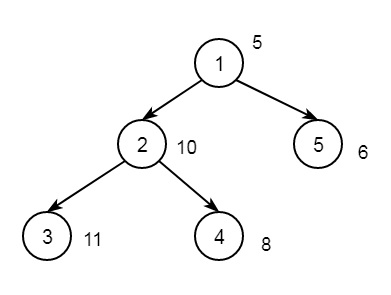# Find the node whose absolute difference with X gives maximum value in C++

Suppose we have a tree, and the weights of all the nodes and an integer x. We have to find the node i, such that |weight[i] - x| is minimum. If the graph is like below, and x = 15Output will be 3. Now for different nodes, it will be like below

Node 1, |5 – 15| = 10

Node 2, |10 – 15| = 5

Node 3, |11 – 15| = 4

Node 4, |8 – 15| = 7

Node 5, |6 – 15| = 9

The idea is simple. We will perform the DFS on the tree, and keep track of the node, whose weighted absolute difference with x gives the minimum value

## Example

#include <iostream>
#include <vector>
#include <cmath>
using namespace std;
int min_value = INT_MAX, x, result;
vector<int> graph;
vector<int> weight(100);
void dfs(int node, int parent) {
if (min_value > abs(weight[node] - x)) {
min_value = abs(weight[node] - x);
result = node;
}
for (int to : graph[node]) {
if (to == parent)
continue;
dfs(to, node);
}
}
int main() {
x = 15;
weight = 5;
weight = 10;
weight = 11;
weight = 8;
weight = 6;
graph.push_back(2);
graph.push_back(3);
graph.push_back(4);
graph.push_back(5);
dfs(1, 1);
cout << "The node number is: " << result;
}

## Output

The node number is: 3